Скачать презентацию ﺑﺴﻢ ﺍﻟﻠﻪ ﺍﻟﺮﺣﻤﻦ ﺍﻟﺮﺣﻴﻢ An-najah national university

044c3d3017901a953441ec0d32a6b68b.ppt

• Количество слайдов: 35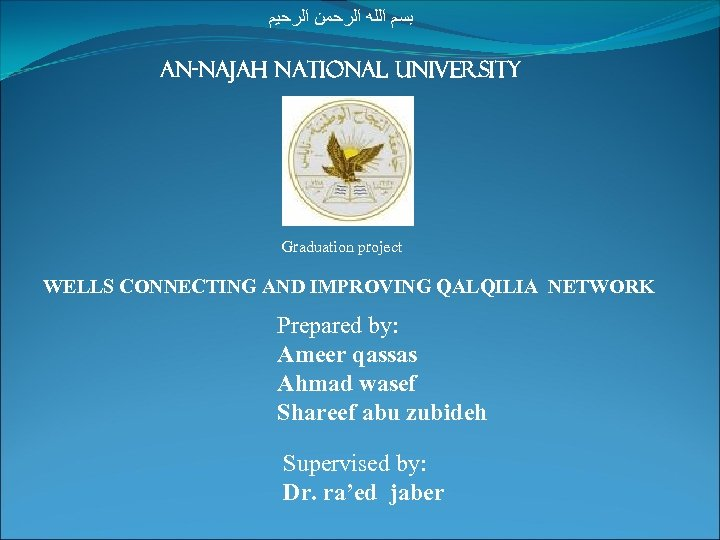ﺑﺴﻢ ﺍﻟﻠﻪ ﺍﻟﺮﺣﻤﻦ ﺍﻟﺮﺣﻴﻢ An-najah national university Graduation project WELLS CONNECTING AND IMPROVING QALQILIA NETWORK Prepared by: Ameer qassas Ahmad wasef Shareef abu zubideh Supervised by: Dr. ra’ed jaberINTRODUCTION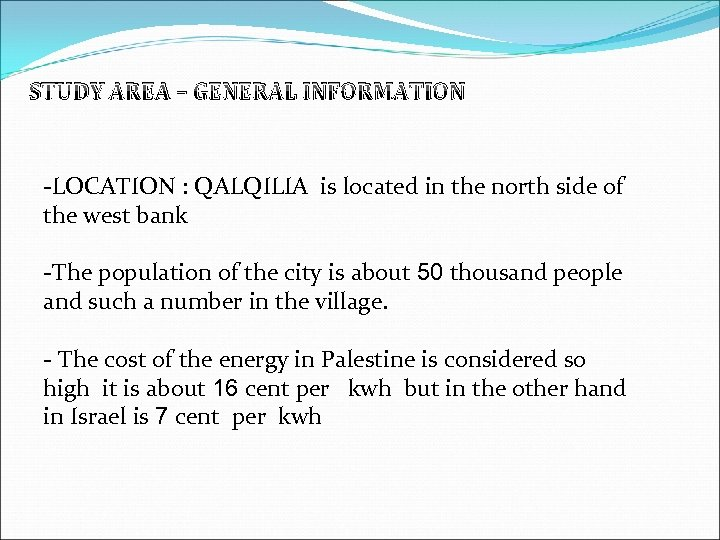STUDY AREA – GENERAL INFORMATION -LOCATION : QALQILIA is located in the north side of the west bank -The population of the city is about 50 thousand people and such a number in the village. - The cost of the energy in Palestine is considered so high it is about 16 cent per kwh but in the other hand in Israel is 7 cent per kwh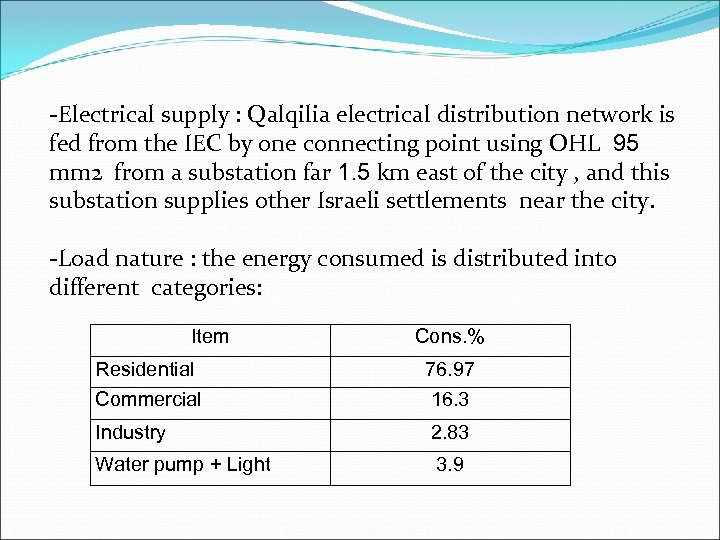-Electrical supply : Qalqilia electrical distribution network is fed from the IEC by one connecting point using OHL 95 mm 2 from a substation far 1. 5 km east of the city , and this substation supplies other Israeli settlements near the city. -Load nature : the energy consumed is distributed into different categories: Item Cons. % Residential Commercial 16. 3 Industry 2. 83 Water pump + Light 76. 97 3. 9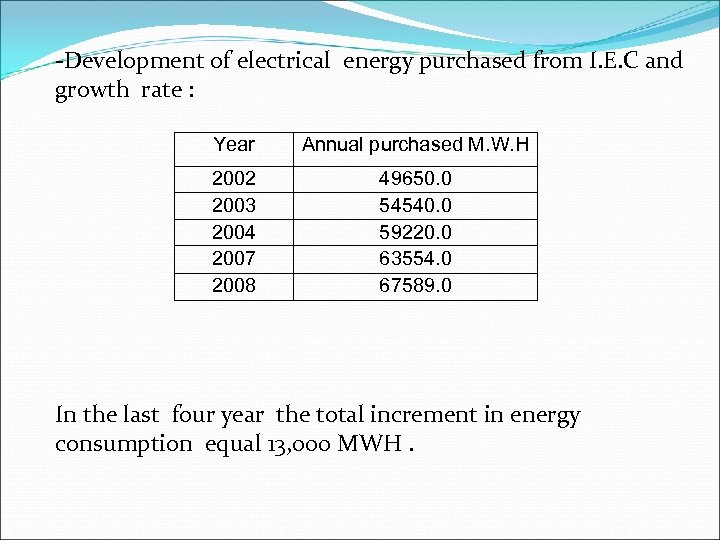-Development of electrical energy purchased from I. E. C and growth rate : Year Annual purchased M. W. H 2002 2003 2004 2007 2008 49650. 0 54540. 0 59220. 0 63554. 0 67589. 0 In the last four year the total increment in energy consumption equal 13, 000 MWH.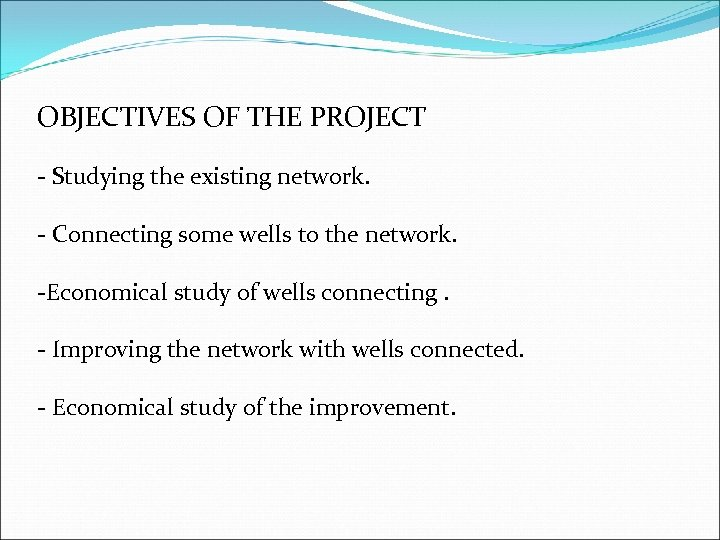OBJECTIVES OF THE PROJECT - Studying the existing network. - Connecting some wells to the network. -Economical study of wells connecting. - Improving the network with wells connected. - Economical study of the improvement.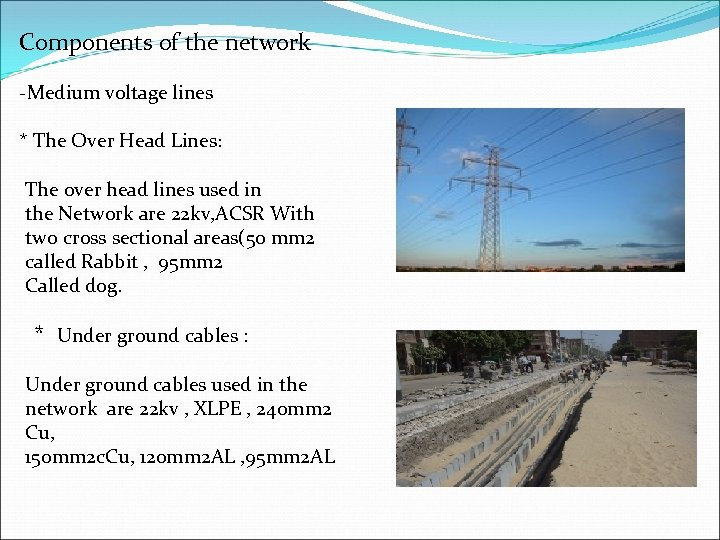Components of the network -Medium voltage lines * The Over Head Lines: The over head lines used in the Network are 22 kv, ACSR With two cross sectional areas(50 mm 2 called Rabbit , 95 mm 2 Called dog. * Under ground cables : Under ground cables used in the network are 22 kv , XLPE , 240 mm 2 Cu, 150 mm 2 c. Cu, 120 mm 2 AL , 95 mm 2 AL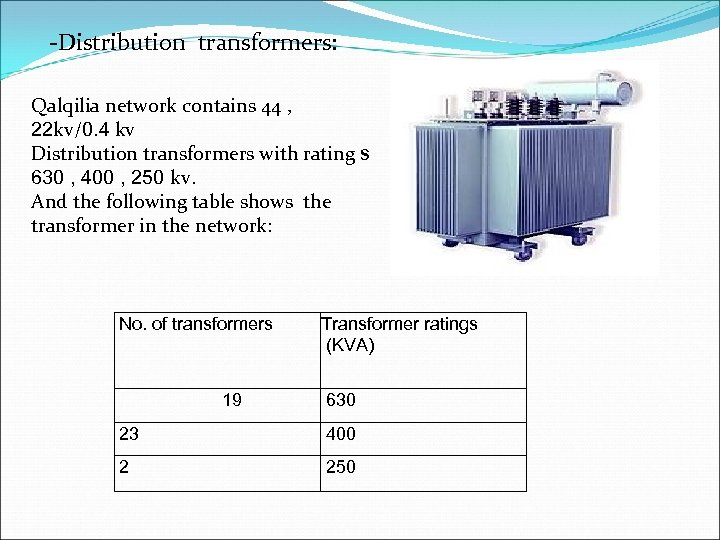-Distribution transformers: Qalqilia network contains 44 , 22 kv/0. 4 kv Distribution transformers with rating s 630 , 400 , 250 kv. And the following table shows the transformer in the network: No. of transformers 19 Transformer ratings (KVA) 630 23 400 2 250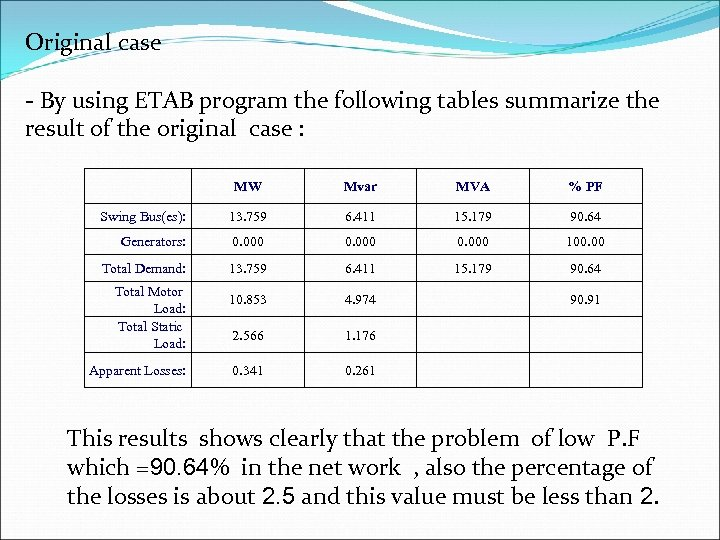Original case - By using ETAB program the following tables summarize the result of the original case : MW Mvar MVA % PF Swing Bus(es): 13. 759 6. 411 15. 179 90. 64 Generators: 0. 000 100. 00 Total Demand: 13. 759 6. 411 15. 179 90. 64 10. 853 4. 974 2. 566 1. 176 0. 341 0. 261 Total Motor Load: Total Static Load: Apparent Losses: 90. 91 This results shows clearly that the problem of low P. F which =90. 64% in the net work , also the percentage of the losses is about 2. 5 and this value must be less than 2.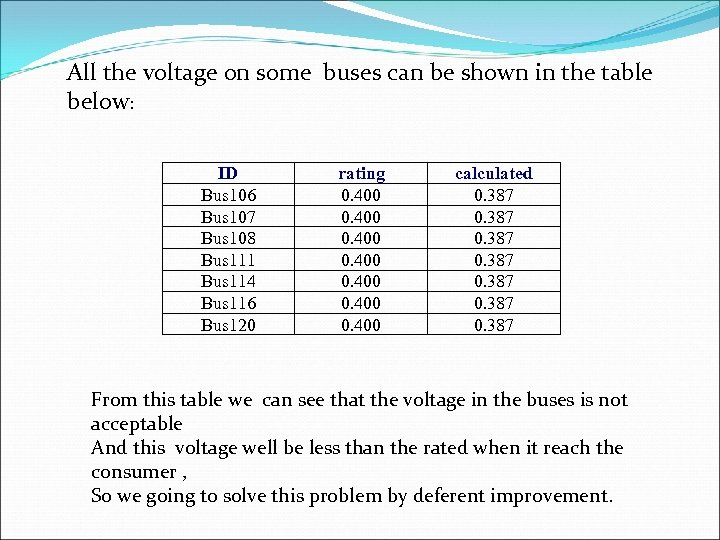All the voltage on some buses can be shown in the table below: ID Bus 106 Bus 107 Bus 108 Bus 111 Bus 114 Bus 116 Bus 120 rating 0. 400 0. 400 calculated 0. 387 0. 387 From this table we can see that the voltage in the buses is not acceptable And this voltage well be less than the rated when it reach the consumer , So we going to solve this problem by deferent improvement.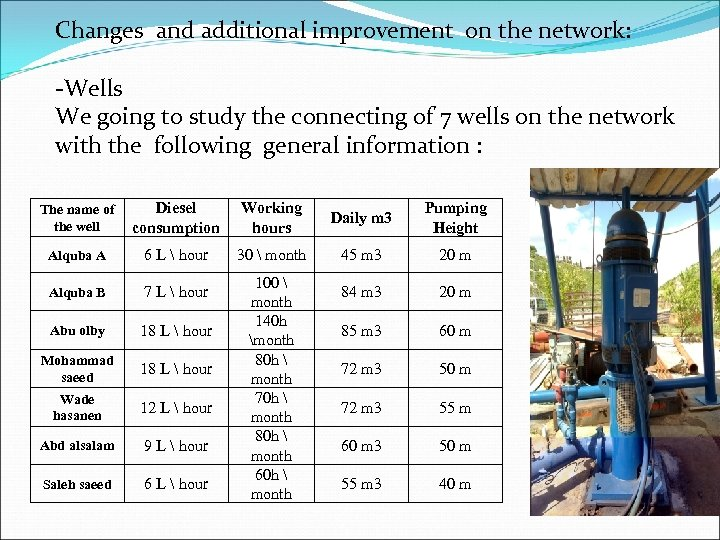Changes and additional improvement on the network: -Wells We going to study the connecting of 7 wells on the network with the following general information : The name of the well Diesel consumption Working hours Daily m 3 Pumping Height Alquba A 6 L hour 30 month 45 m 3 20 m Alquba B 7 L hour 84 m 3 20 m Abu olby 18 L hour 85 m 3 60 m Mohammad saeed 18 L hour 72 m 3 50 m Wade hasanen 12 L hour 72 m 3 55 m Abd alsalam 9 L hour 60 m 3 50 m Saleh saeed 6 L hour 55 m 3 40 m 100 month 140 h month 80 h month 70 h month 80 h month 60 h month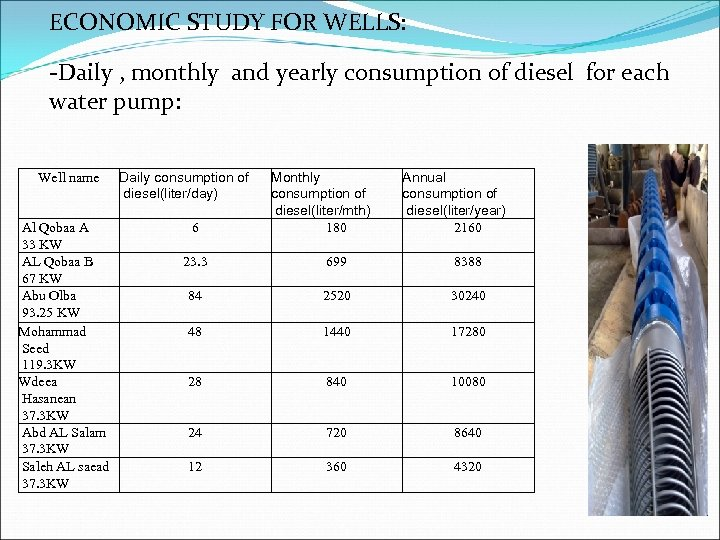ECONOMIC STUDY FOR WELLS: -Daily , monthly and yearly consumption of diesel for each water pump: Well name Al Qobaa A 33 KW AL Qobaa B 67 KW Abu Olba 93. 25 KW Mohammad Seed 119. 3 KW Wdeea Hasanean 37. 3 KW Abd AL Salam 37. 3 KW Saleh AL saead 37. 3 KW Daily consumption of diesel(liter/day) 6 Monthly consumption of diesel(liter/mth) 180 Annual consumption of diesel(liter/year) 2160 23. 3 699 8388 84 2520 30240 48 1440 17280 28 840 10080 24 720 8640 12 360 4320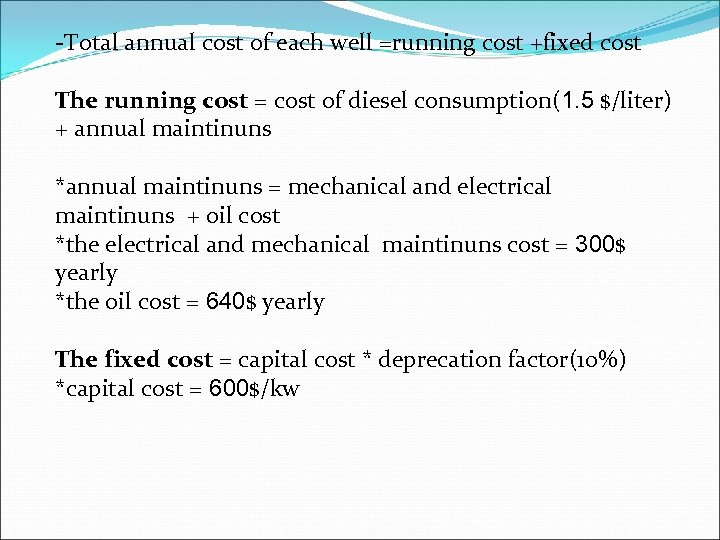-Total annual cost of each well =running cost +fixed cost The running cost = cost of diesel consumption(1. 5 \$/liter) + annual maintinuns *annual maintinuns = mechanical and electrical maintinuns + oil cost *the electrical and mechanical maintinuns cost = 300\$ yearly *the oil cost = 640\$ yearly The fixed cost = capital cost * deprecation factor(10%) *capital cost = 600\$/kw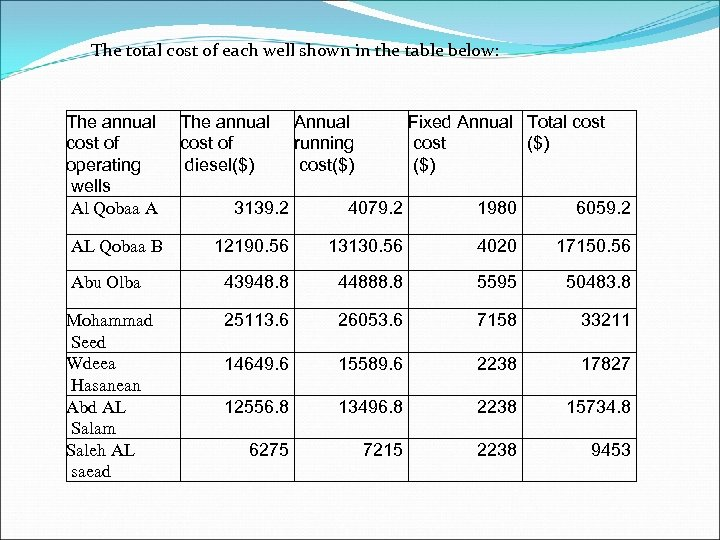The total cost of each well shown in the table below: The annual cost of operating wells Al Qobaa A The annual cost of diesel(\$) Annual running cost(\$) Fixed Annual Total cost (\$) 3139. 2 4079. 2 1980 6059. 2 12190. 56 13130. 56 4020 17150. 56 Abu Olba 43948. 8 44888. 8 5595 50483. 8 Mohammad Seed Wdeea Hasanean Abd AL Salam Saleh AL saead 25113. 6 26053. 6 7158 33211 14649. 6 15589. 6 2238 17827 12556. 8 13496. 8 2238 15734. 8 6275 7215 2238 9453 AL Qobaa B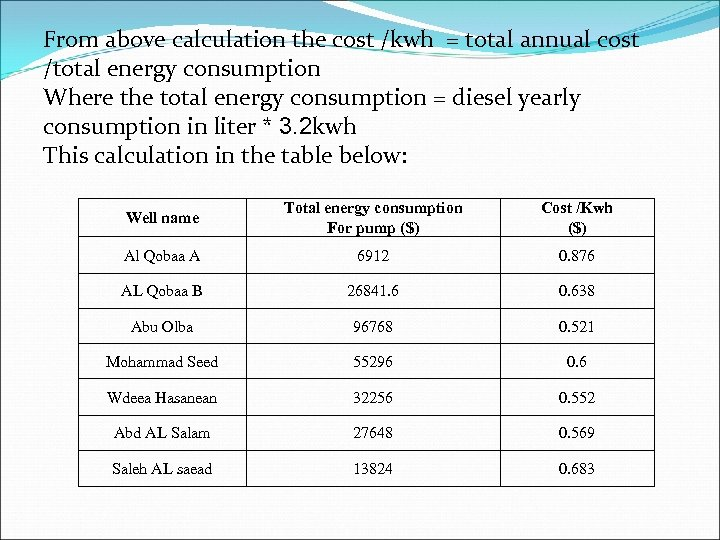From above calculation the cost /kwh = total annual cost /total energy consumption Where the total energy consumption = diesel yearly consumption in liter * 3. 2 kwh This calculation in the table below: Well name Total energy consumption For pump (\$) Cost /Kwh (\$) Al Qobaa A 6912 0. 876 AL Qobaa B 26841. 6 0. 638 Abu Olba 96768 0. 521 Mohammad Seed 55296 0. 6 Wdeea Hasanean 32256 0. 552 Abd AL Salam 27648 0. 569 Saleh AL saead 13824 0. 683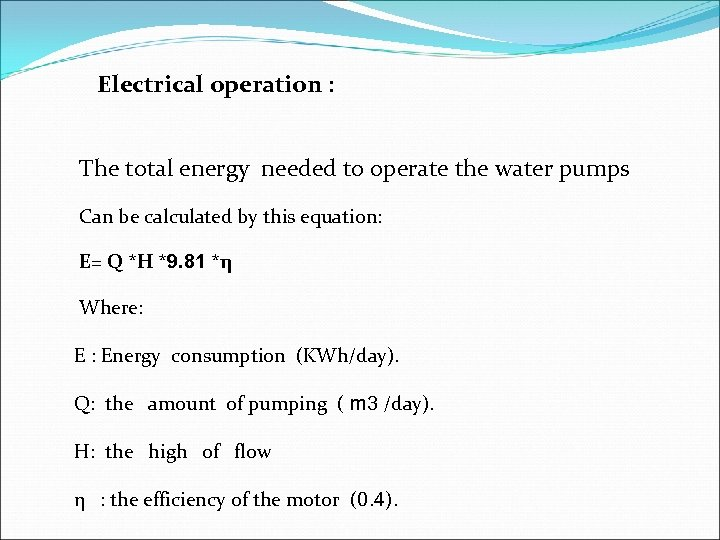Electrical operation : The total energy needed to operate the water pumps Can be calculated by this equation: E= Q *H *9. 81 *η Where: E : Energy consumption (KWh/day). Q: the amount of pumping ( m 3 /day). H: the high of flow η : the efficiency of the motor (0. 4).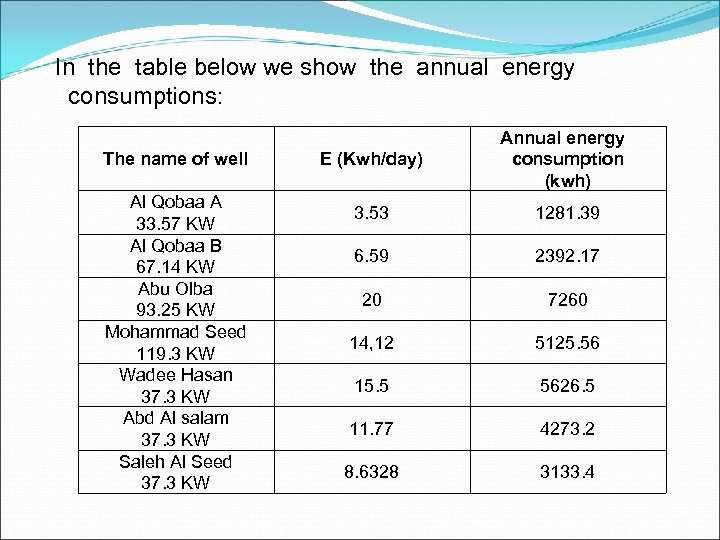In the table below we show the annual energy consumptions: The name of well Al Qobaa A 33. 57 KW Al Qobaa B 67. 14 KW Abu Olba 93. 25 KW Mohammad Seed 119. 3 KW Wadee Hasan 37. 3 KW Abd Al salam 37. 3 KW Saleh Al Seed 37. 3 KW E (Kwh/day) Annual energy consumption (kwh) 3. 53 1281. 39 6. 59 2392. 17 20 7260 14, 12 5125. 56 15. 5 5626. 5 11. 77 4273. 2 8. 6328 3133. 4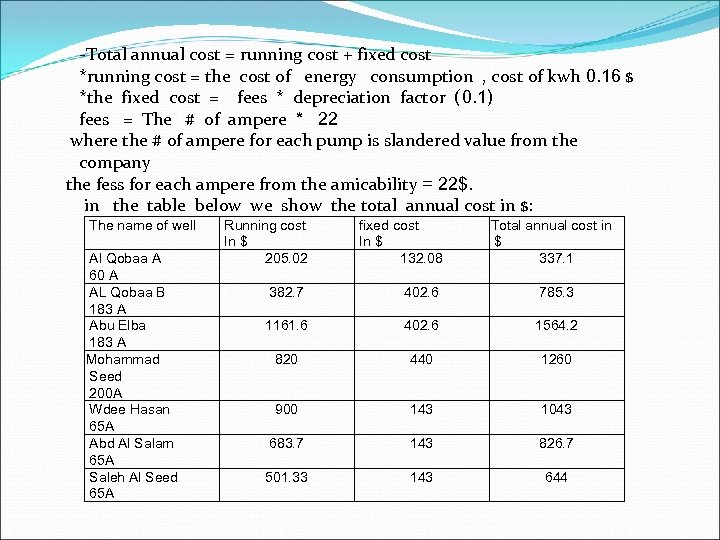-Total annual cost = running cost + fixed cost *running cost = the cost of energy consumption , cost of kwh 0. 16 \$ *the fixed cost = fees * depreciation factor ( 0. 1) fees = The # of ampere * 22 where the # of ampere for each pump is slandered value from the company the fess for each ampere from the amicability = 22\$. in the table below we show the total annual cost in \$: The name of well Al Qobaa A 60 A AL Qobaa B 183 A Abu Elba 183 A Mohammad Seed 200 A Wdee Hasan 65 A Abd Al Salam 65 A Saleh Al Seed 65 A Running cost In \$ 205. 02 fixed cost In \$ 132. 08 Total annual cost in \$ 337. 1 382. 7 402. 6 785. 3 1161. 6 402. 6 1564. 2 820 440 1260 900 143 1043 683. 7 143 826. 7 501. 33 143 644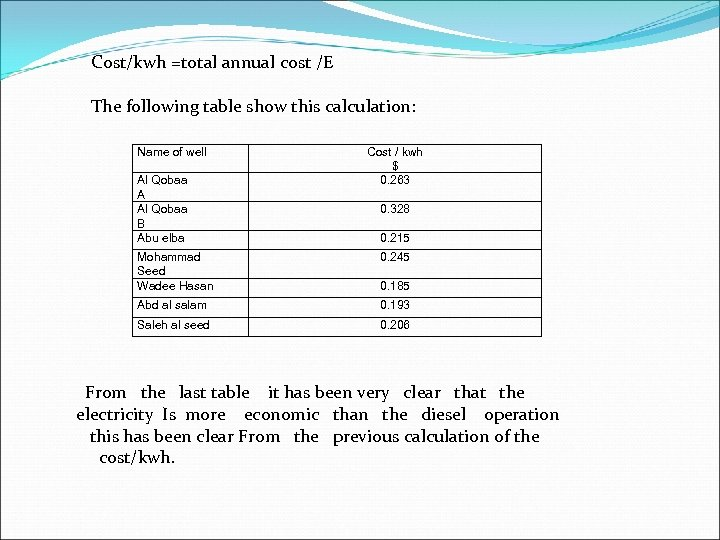Cost/kwh =total annual cost /E The following table show this calculation: Name of well Al Qobaa A Al Qobaa B Abu elba Cost / kwh \$ 0. 263 0. 328 0. 215 Mohammad Seed Wadee Hasan 0. 245 Abd al salam 0. 193 Saleh al seed 0. 206 0. 185 From the last table it has been very clear that the electricity Is more economic than the diesel operation this has been clear From the previous calculation of the cost/kwh.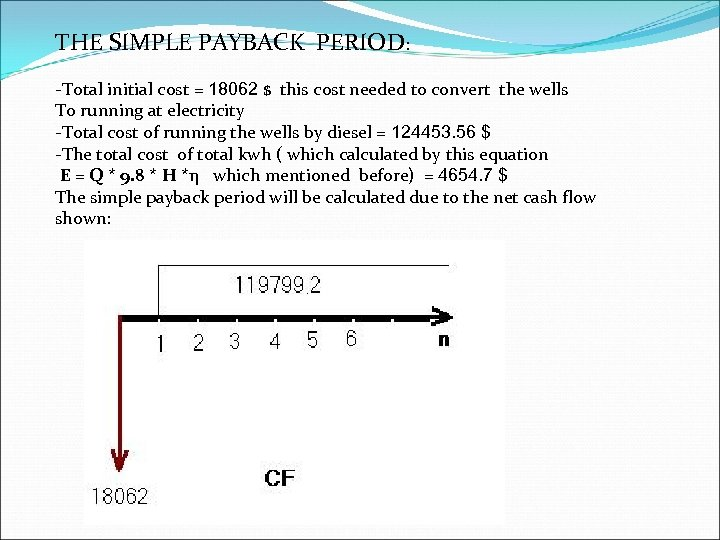THE SIMPLE PAYBACK PERIOD: -Total initial cost = 18062 \$ this cost needed to convert the wells To running at electricity -Total cost of running the wells by diesel = 124453. 56 \$ -The total cost of total kwh ( which calculated by this equation E = Q * 9. 8 * H *η which mentioned before) = 4654. 7 \$ The simple payback period will be calculated due to the net cash flow shown: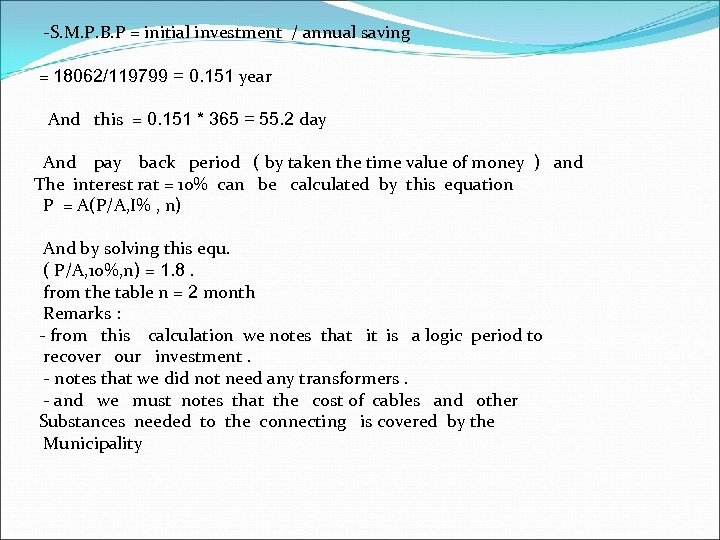-S. M. P. B. P = initial investment / annual saving = 18062/119799 = 0. 151 year And this = 0. 151 * 365 = 55. 2 day And pay back period ( by taken the time value of money ) and The interest rat = 10% can be calculated by this equation P = A(P/A, I% , n) And by solving this equ. ( P/A, 10%, n) = 1. 8. from the table n = 2 month Remarks : - from this calculation we notes that it is a logic period to recover our investment. - notes that we did not need any transformers. - and we must notes that the cost of cables and other Substances needed to the connecting is covered by the Municipality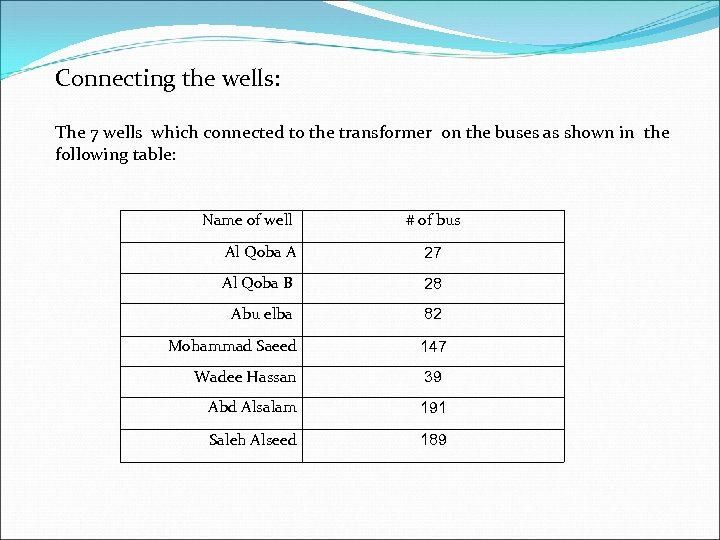Connecting the wells: The 7 wells which connected to the transformer on the buses as shown in the following table: Name of well # of bus Al Qoba A 27 Al Qoba B 28 Abu elba 82 Mohammad Saeed 147 Wadee Hassan 39 Abd Alsalam 191 Saleh Alseed 189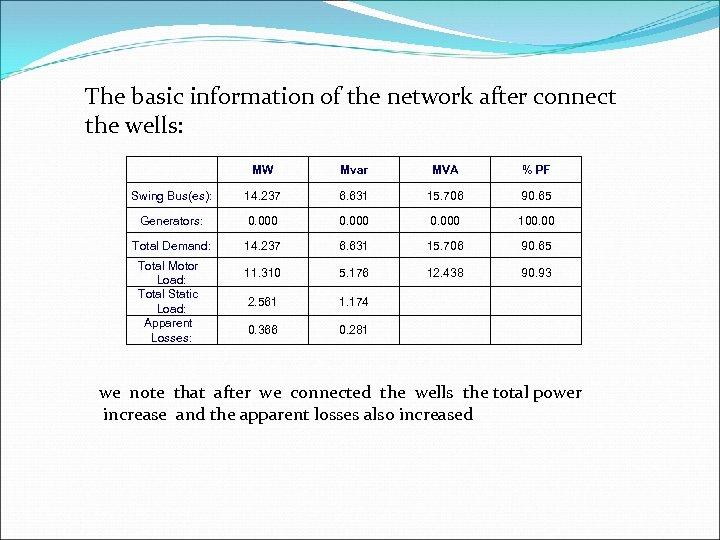The basic information of the network after connect the wells: MW Mvar MVA % PF Swing Bus(es): 14. 237 6. 631 15. 706 90. 65 Generators: 0. 000 100. 00 Total Demand: 14. 237 6. 631 15. 706 90. 65 11. 310 5. 176 12. 438 90. 93 2. 561 1. 174 0. 366 0. 281 Total Motor Load: Total Static Load: Apparent Losses: we note that after we connected the wells the total power increase and the apparent losses also increased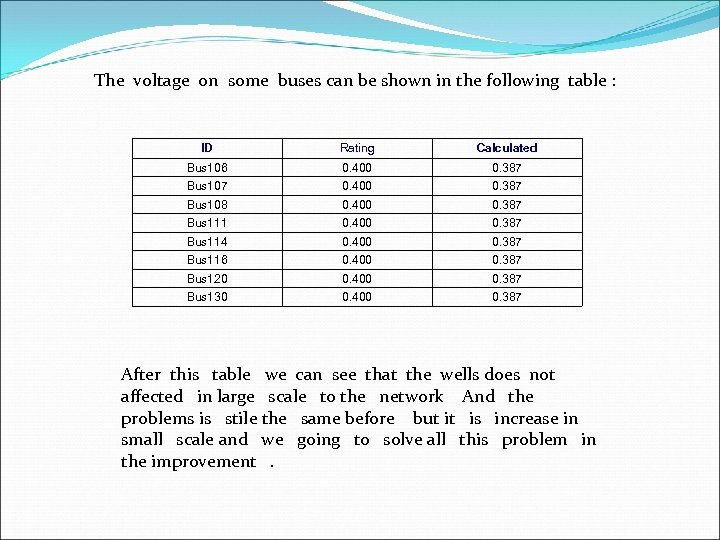The voltage on some buses can be shown in the following table : ID Rating Calculated Bus 106 0. 400 0. 387 Bus 107 0. 400 0. 387 Bus 108 0. 400 0. 387 Bus 111 0. 400 0. 387 Bus 114 0. 400 0. 387 Bus 116 0. 400 0. 387 Bus 120 0. 400 0. 387 Bus 130 0. 400 0. 387 After this table we can see that the wells does not affected in large scale to the network And the problems is stile the same before but it is increase in small scale and we going to solve all this problem in the improvement .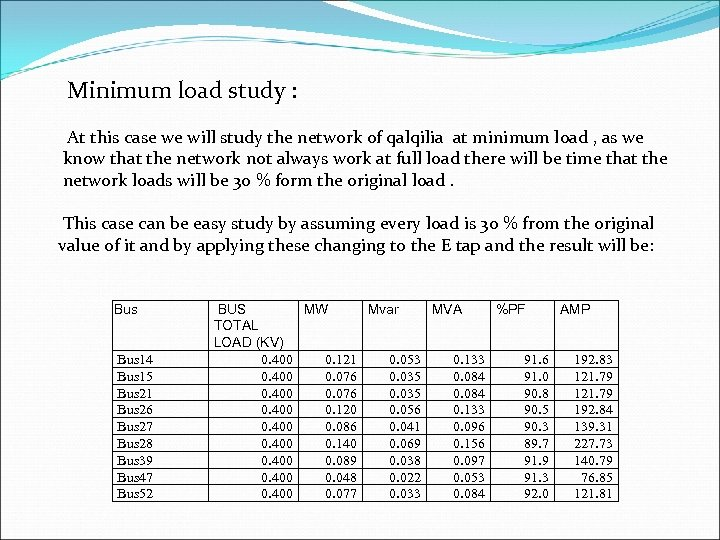Minimum load study : At this case we will study the network of qalqilia at minimum load , as we know that the network not always work at full load there will be time that the network loads will be 30 % form the original load. This case can be easy study by assuming every load is 30 % from the original value of it and by applying these changing to the E tap and the result will be: Bus 14 Bus 15 Bus 21 Bus 26 Bus 27 Bus 28 Bus 39 Bus 47 Bus 52 BUS MW Mvar MVA %PF AMP TOTAL LOAD (KV) 0. 400 0. 121 0. 053 0. 133 91. 6 192. 83 0. 400 0. 076 0. 035 0. 084 91. 0 121. 79 0. 400 0. 076 0. 035 0. 084 90. 8 121. 79 0. 400 0. 120 0. 056 0. 133 90. 5 192. 84 0. 400 0. 086 0. 041 0. 096 90. 3 139. 31 0. 400 0. 140 0. 069 0. 156 89. 7 227. 73 0. 400 0. 089 0. 038 0. 097 91. 9 140. 79 0. 400 0. 048 0. 022 0. 053 91. 3 76. 85 0. 400 0. 077 0. 033 0. 084 92. 0 121. 81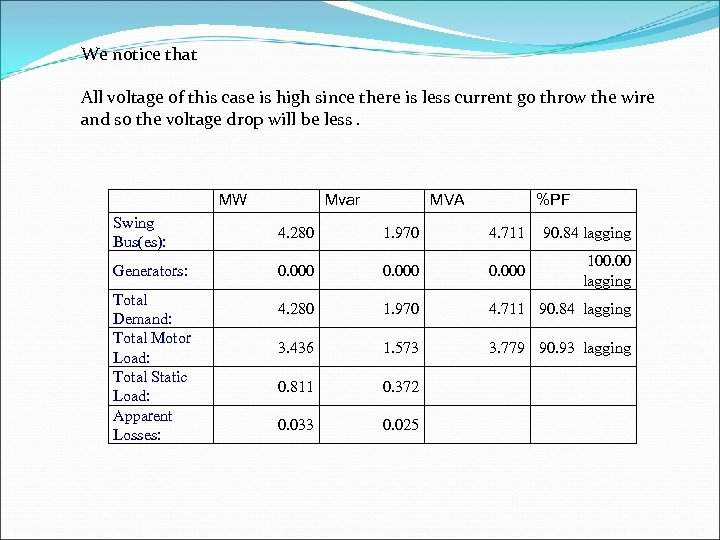We notice that All voltage of this case is high since there is less current go throw the wire and so the voltage drop will be less. MW Mvar MVA %PF Swing Bus(es): 4. 280 1. 970 4. 711 90. 84 lagging Generators: 0. 000 100. 00 lagging 4. 280 1. 970 4. 711 90. 84 lagging 3. 436 1. 573 3. 779 90. 93 lagging 0. 811 0. 372 0. 033 0. 025 Total Demand: Total Motor Load: Total Static Load: Apparent Losses: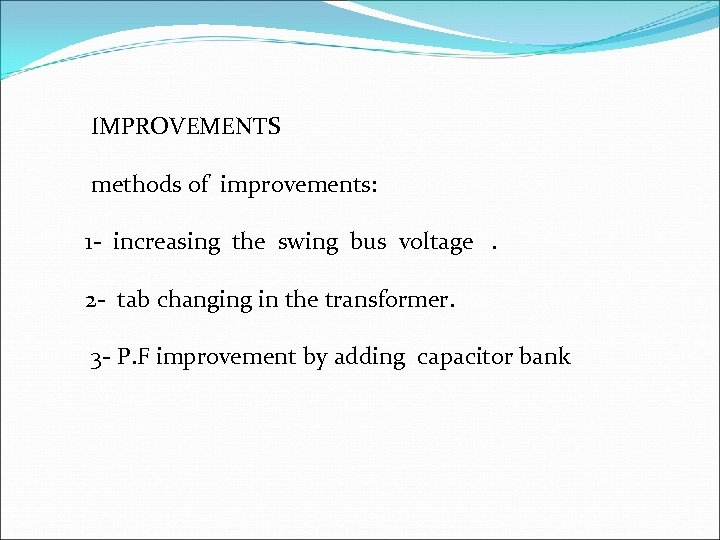IMPROVEMENTS methods of improvements: 1 - increasing the swing bus voltage . 2 - tab changing in the transformer. 3 - P. F improvement by adding capacitor bank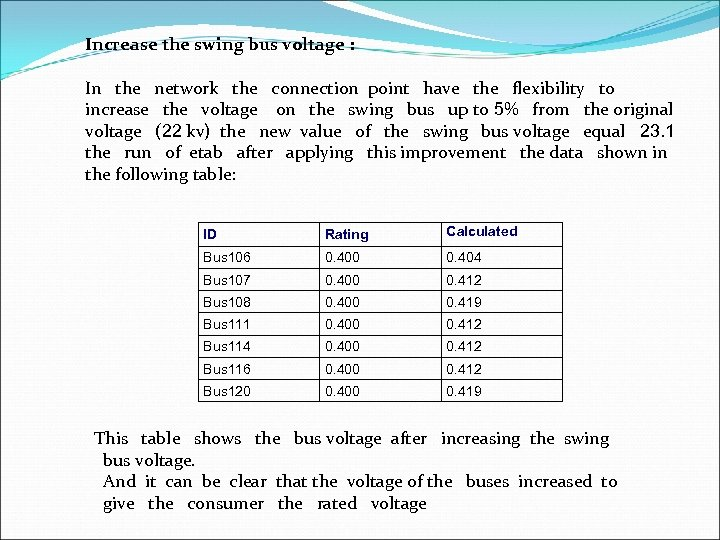Increase the swing bus voltage : In the network the connection point have the flexibility to increase the voltage on the swing bus up to 5% from the original voltage (22 kv) the new value of the swing bus voltage equal 23. 1 the run of etab after applying this improvement the data shown in the following table: ID Rating Calculated Bus 106 0. 400 0. 404 Bus 107 0. 400 0. 412 Bus 108 0. 400 0. 419 Bus 111 0. 400 0. 412 Bus 114 0. 400 0. 412 Bus 116 0. 400 0. 412 Bus 120 0. 400 0. 419 This table shows the bus voltage after increasing the swing bus voltage. And it can be clear that the voltage of the buses increased to give the consumer the rated voltage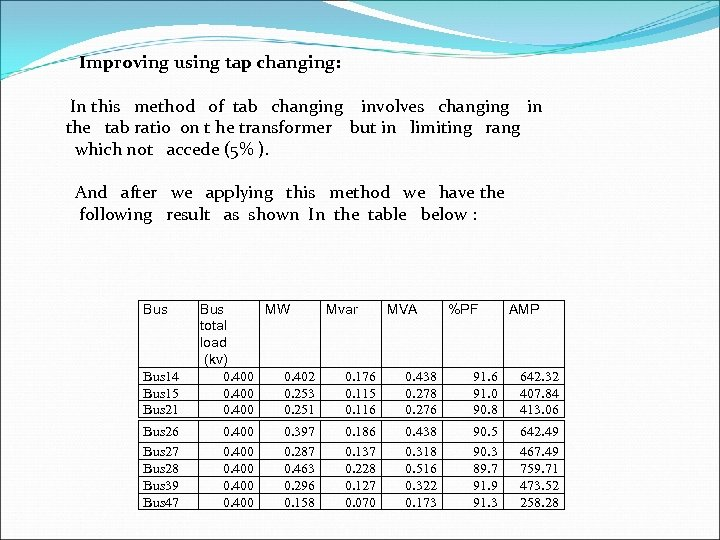Improving using tap changing: In this method of tab changing involves changing in the tab ratio on t he transformer but in limiting rang which not accede (5% ). And after we applying this method we have the following result as shown In the table below : Bus 14 Bus 15 Bus 21 Bus 26 Bus 27 Bus 28 Bus 39 Bus 47 Bus MW Mvar MVA %PF AMP total load (kv) 0. 400 0. 402 0. 176 0. 438 91. 6 642. 32 0. 400 0. 253 0. 115 0. 278 91. 0 407. 84 0. 400 0. 251 0. 116 0. 276 90. 8 413. 06 0. 400 0. 397 0. 287 0. 463 0. 296 0. 158 0. 186 0. 137 0. 228 0. 127 0. 070 0. 438 0. 318 0. 516 0. 322 0. 173 90. 5 90. 3 89. 7 91. 9 91. 3 642. 49 467. 49 759. 71 473. 52 258. 28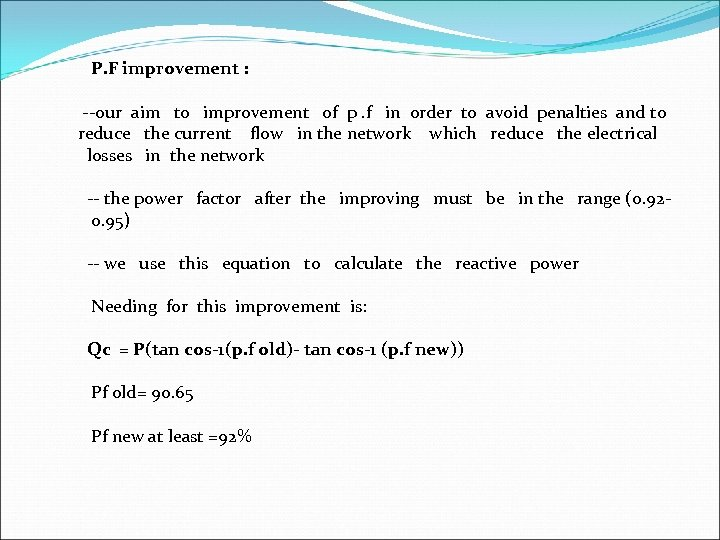P. F improvement : --our aim to improvement of p. f in order to avoid penalties and to reduce the current flow in the network which reduce the electrical losses in the network -- the power factor after the improving must be in the range (0. 92 - 0. 95) -- we use this equation to calculate the reactive power Needing for this improvement is: Qc = P(tan cos-1(p. f old)- tan cos-1 (p. f new)) Pf old= 90. 65 Pf new at least =92%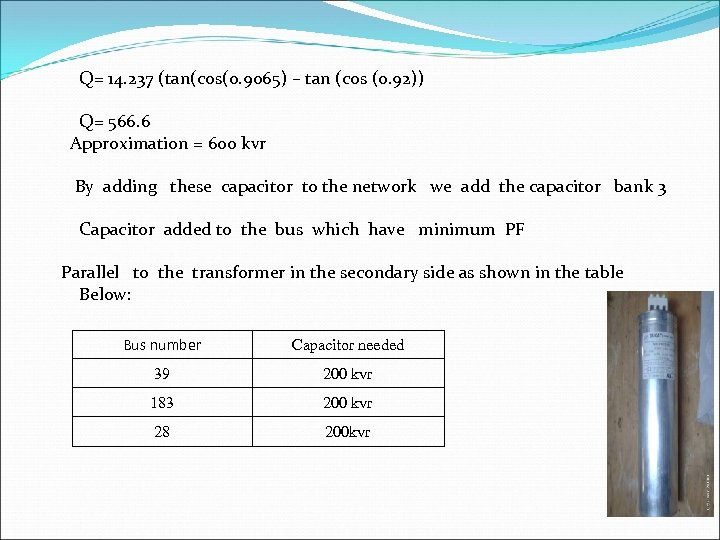Q= 14. 237 (tan(cos(0. 9065) – tan (cos (0. 92)) Q= 566. 6 Approximation = 600 kvr By adding these capacitor to the network we add the capacitor bank 3 Capacitor added to the bus which have minimum PF Parallel to the transformer in the secondary side as shown in the table Below: Bus number Capacitor needed 39 200 kvr 183 200 kvr 28 200 kvr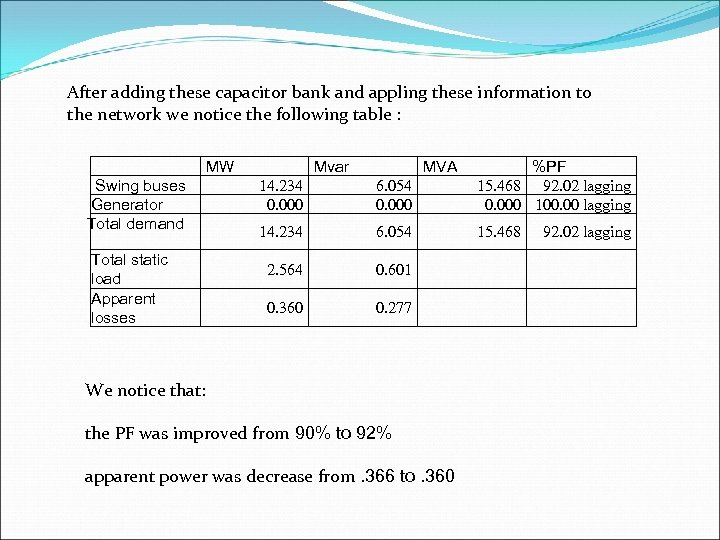After adding these capacitor bank and appling these information to the network we notice the following table : MW Swing buses Generator Total demand Total static load Apparent losses Mvar MVA 14. 234 0. 000 6. 054 0. 000 %PF 15. 468 92. 02 lagging 0. 000 100. 00 lagging 14. 234 6. 054 15. 468 2. 564 0. 601 0. 360 0. 277 We notice that: the PF was improved from 90% to 92% apparent power was decrease from. 366 to. 360 92. 02 lagging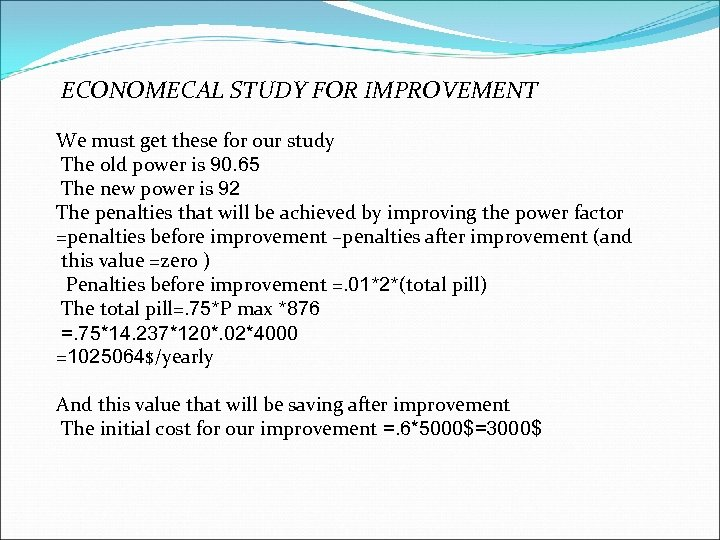ECONOMECAL STUDY FOR IMPROVEMENT We must get these for our study The old power is 90. 65 The new power is 92 The penalties that will be achieved by improving the power factor =penalties before improvement –penalties after improvement (and this value =zero ) Penalties before improvement =. 01*2*(total pill) The total pill=. 75*P max *876 =. 75*14. 237*120*. 02*4000 =1025064\$/yearly And this value that will be saving after improvement The initial cost for our improvement =. 6*5000\$=3000\$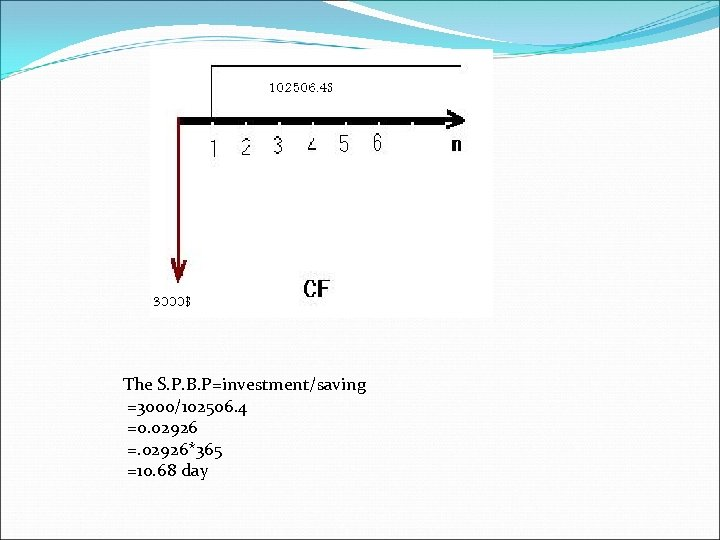The S. P. B. P=investment/saving =3000/102506. 4 =0. 02926 =. 02926*365 =10. 68 day